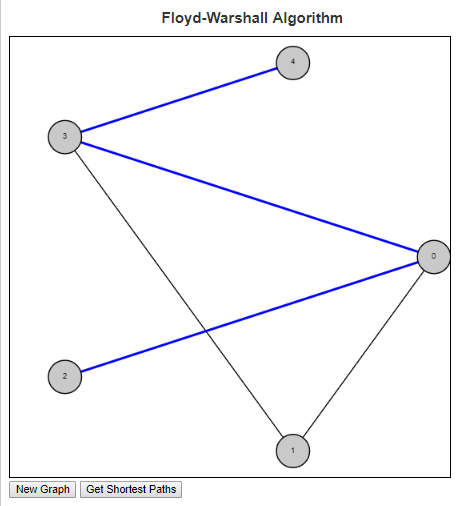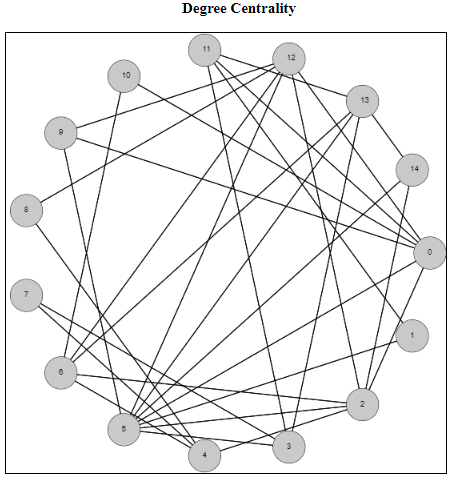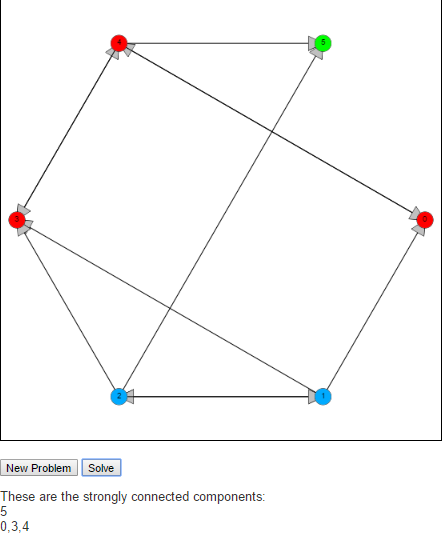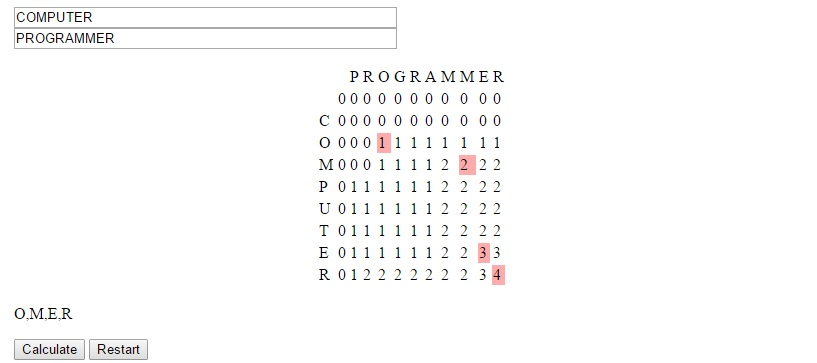# Set Partition Problems

This is my first post of 2019 and my first post in a while. There was one posted a few months ago, but not really geared towards the algorithms and learning focus of the site. I have been doing a lot of coding in my spare time, but honestly life has just gotten in the way. Its not a bad thing, but life is life and sometimes I have to prioritize the things. In particular, I have been having ideas and actually coding things up but the time it takes to clean up code, write a blog entry and finding a nice way to visualize these things has been something that I haven’t been able to really focus on as much as I’ve wanted to.

That said, I want to talk to you about the Set Partition Problem today. You can go to Wikipedia to get more information about this problem, but I will give you a brief introduction to it and then talk about two different approaches to it. The problem assumes that we are given as input a (multi)-set S. The reason we say it is a multi-set and not a simple set is because we can have the same element appear multiple times in the set. So if S1 = {2} and S2 = {2, 2}, then although as sets they are both equal to the set {2} = S1, as multi-sets allow for multiple instances of an element. The elements of S are assumed to be positive integers.

So given this multi-set S, we ask the question of can the elements of S be divided into two smaller multi-sets, C1 and C2 where

• C1 [union] C2 = S
• C1 [intersect] C2 = [empty set]
• [Sigma]_[x in C1] = [Sigma]_[x in C2].C1 [union] C2 = S, C1 [intersect] C2 = [empty set], and [Sigma]_[x in C1] = [Sigma]_[x in C2]

The first two bullets above say that the sets C1 and C2 form a partition of S. The third bullet says that the sums of the elements in the two children multi-sets are equal.

This problem is known to be NP Complete. This means that it is one of the more difficult decision problems. Because of this finding an algorithm that solves this problem exactly will generally take a long running time. And finding an algorithm that runs quickly will more than likely be incorrect in some instances.

I will show you two approaches to this problem. One is based on Dynamic Programming (DP) and one is based on Greedy Algorithms. The DP version solves the problem exactly but has a slow running time. The greedy algorithm is fast but is not guaranteed to always give the correct answer.

Dynamic Programming is based on the principle of optimality. This says that in order to have a correct solution to the overall problem, we need optimal solutions to all subproblems of this problem. This is done by keeping track of a table which can be used for looking up these subproblems and the optimal solutions to these subproblems.

For this problem, we will build a table where the rows represent the final sums and columns represent subsets of the given multi-set (containing the first 0…n elements). The question we are repeatedly asking is “can we find a subset of the set in this column whose sum is exactly the given rowsum?” Here is an example of the DP algorithm on the multi-set {5, 6, 5, 6, 7}.

Greedy Algorithms are generally based on sorting the elements based on some principle and using that to try to answer the underlying question. The main problem with this approach is that it is very short sided because they do not look at the overall picture. Such algorithms are known for finding local optima that are not always globally optimal. The benefit to these problems though is that they are generally easier to code and easier to understand.

For the Set Partition Problem , the Greedy approach is to sort elements in descending order. Once this is done, the goal is to keep two subsets while iterating through the array, adding the element to the smaller of the two sets whenever possible.

For more examples, check out Set Partition Problems

# Floyd-Warshall Shortest PathsThe Floyd Warshall algorithm is an all pairs shortest paths algorithm. This can be contrasted with algorithms like Dijkstra’s which give the shortest paths from a single node to all other nodes in the graph.

Floyd Warshall’s algorithm works by considering first the edge set of the graph. This is the set of all paths of the graph through one edge. Node pairs that are connected to one another through an edge will have their shortest path set to the length of that edge, while all other node pairs will have their shortest path set to infinity. The program then runs through every triplet of nodes (i, j, k) and checks if the path from i to k and the path from k to j is shorter than the current path from i to j. If so, then the distance and the path is updated.

So lets consider an example on the graph in the image above. The edge set of this graph is E = {(0, 1), (0, 2), (0, 3), (1, 3), (3, 4)}. So our initial table is:

 0 1 2 3 4 0 inf (0, 1) (0, 2) (0, 3) inf 1 (0, 1) inf inf (1, 3) inf 2 (0, 2) inf inf inf inf 3 (0, 3) (1, 3) inf inf (3, 4) 4 inf inf inf (3, 4) inf

As we look to update the paths, we first look for routes that go through node 0:

Because node 0 connects to both node 1 and node 2, but node 1 does not connect to node 2, we have the following truth holding in the matrix above:
cost(0, 1) + cost(0, 2) < cost(1, 2), so we can update the shortest path from node 1 to node 2 to be (1, 0, 2).

Because node 0 connects to both node 2 and node 3, but node 2 does not connect to node 3, we have the following truth holding in the matrix above:
cost(0, 2) + cost(0, 3) < cost(2, 3), so we can update the shortest path from node 2 to node 3 to be (2, 0, 3).

Because node 3 connects to both node 0 and node 4, but node 0 does not connect to node 4, we have the following truth holding in the matrix above:
cost(0, 3) + cost(3, 4) < cost(0, 4), so we can update the shortest path from node 0 to node 4 to be (0, 3, 4).

Because node 3 connects to both node 1 and node 4, but node 1 does not connect to node 4, we have the following truth holding in the matrix above:
cost(1, 3) + cost(3, 4) < cost(1, 4), so we can update the shortest path from node 1 to node 4 to be (1, 3, 4).

Because node 3 connects to both node 2 and node 4, but node 2 does not connect to node 4, we have the following truth now holding:
cost(2, 3) + cost(3, 4) < cost(2, 4), so we can update the shortest path from node 2 to node 4 to be (2, 0, 3, 4).

The final table giving the list of shortest paths from every node to every other node is given below.

 0 1 2 3 4 0 inf (0, 1) (0, 2) (0, 3) (0, 3, 4) 1 (0, 1) inf (1, 0, 2) (1, 3) (1, 3, 4) 2 (0, 2) (1, 0, 2) inf (2, 0, 3) (2, 0, 3, 4) 3 (0, 3) (1, 3) (2, 0, 3) inf (3, 4) 4 (0, 3, 4) (1, 3, 4) (2, 0, 3, 4) (3, 4) inf

To see more examples and to help answer questions, check out the script in my examples section on the Floyd-Warshall algorithm

# Degree Centrality of a GraphI wanted to spend some time on centrality measures of a graph. These are measurements of how important each node (or edge) is to the overall graph. But how do we define, or determine, importance? There is no unique way to answer this question, so there are varying metrics for measuring centrality. Which one you choose depends on several factors including how many other nodes of the graph are included, as well as the run time of the metrics you’re considering.

I have just published a script focusing on the degree centrality metric. The degree centrality metric is called a “walk metric” because it determines how important a node is by how many other nodes that can be reached by walks of up to a certain length. Lets look at the definition of the degree of a node to see if we can understand why it is called a walk metric.

In an undirected graph G = (V, E), the degree of a node u [in] V is the |{v | (u, v) [in] E}|. This is the size of the set of nodes that are connected to node u via a single edge. Another way of describing a single edge is a walk of length one. So the degree metric measures the importance of a node by the number of unique walks of length one.

The normalized degree centrality of a node v in a graph G = (V,E) measures how many nodes are connected to the node v, compared to the maximum possible number of edges that can be connected to this node. Because we are dealing with simple undirected graphs (at most a single edge between any two distinct vertices), this maximum possible number will always be |V – 1|. So the normalized degree can be calculated by dividing the degree of the node (the number of nodes it is connected to) by |V – 1|.

So for the example above, the node 0 has degree 6 because it is connected to nodes 2, 5, 9, 10, 11, and 12. There are 15 total nodes in this graph, so to calculate the normalized degree centrality of the node 0, it will be 6 / 14, which rounds to 0.428571.

To see more examples and to help answer questions, check out the script in my examples section on degree centrality

# Tarjan’s Strongly Connected Components AlgorithmA strongly connected component of a graph is a subgraph S of G where every pair of nodes, u and v in S there is a path from u to v and a path from v to u.

To find these strongly connected components we implement Tarjan’s algorithm. The idea behind Tarjan’s algorithm is to begin by running a depth first search from an arbitrary node in the graph, labeling nodes reachable from this start node in the order they are reached. The algorithm is also interested in the “oldest” node that could be reached by a given node. This is indicated by the keeping track of the lowest label that can be reached from that node. We will call the first property label(v) and the second lowlink(v).

When the algorithm starts label(v) is the same as lowlink(v) whenever a node is discovered. As the algorithm is executed, the DFS is being run on each discovered node, which in turn updates the lowlink(v) property telling of (older) nodes that can be reached. If an older node can be reached, then we update lowlink. If we reach a node that cannot connect to any older nodes after the DFS call, i.e if label(v) is the same a lowlink(v), then this means that this node does not have a path to any node with a lower label. So this node will be the first node of a new strongly connected component.

Feel free to check it out an let me know what you think in the comments below.

# Longest Common Subsequence

Suppose you and I each had an ordered list of items and we were interested in comparing how similar those lists are. One calculation we can perform on these two strings is the Longest Common Subsequence. A sequence X is an ordered list of elements <x1, …, xn>. A subsequence Z is another sequence where (1) Each element of Z is also an element of X and (2) The elements of Z occur in the same order (in Z) as they do in X.

Note that we do not say that the elements of Z need to be a continuous block of elements. If this were true we would be defining a substring. So as an example, suppose we have as an initial string,
X = C, O, M, P, U, T, E, R.
Then the following are all subsequences:
Z1 = C, M, U, T, R
Z2 = C, O, M, P
Z3 = U, T, E, R
Z4 = O, P, T, E

I will note that Z2 and Z3 are also substrings since they contain continuous sets of characters.

The length of a substring is simply the number of characters it contains. So X has length 8, Z1 has length 5, Z2, Z3 and Z4 have length 4.

Suppose now that we had a second string, Y = P, R, O, G, R, A, M, M, E, R and are interested in the longest common subsequence between the two. We can do that by observing that there is a bit of recursion going on with this question. What I mean by that is that asking the question of “What is the longest common subsequence between X and Y” is the same as asking “What is the longest common subsequence between X and Y once we have seen 8 characters of X and 10 characters of Y”

There are three possible ways to answer this question.

If X<sub>8</sub> is not equal to Y<sub>10</sub>, then the answer to this will be the same as the maximum of the pair X<sub>7</sub>, Y<sub>10</sub> and the pair X<sub>8</sub>, Y<sub>9</sub>.
If we reach a situation where we reach the beginning of either string, we are forced to answer 0 to that question.

Then the function has the following look:

LCS(Xi, Yj) =
 0, if i is 0 or j is 0 1 + LCS(Xi-1, Yj-1) if Xi equals Yj max(LCS(Xi-1, Yj), LCS(Xi, Yj-1))

Below is a table showing how we would solve the problem mentioned.The strategy used to devise this concept is called dynamic programming. It is useful we can solve larger problems by solving overlapping subproblems, as was the case here. In this situation we generally can store the data in a table form and avoid re-solving subproblems for which many larger problems will be dependent.

You can see this algorithm in action at LEARNINGlover.com: Longest Common Subsequece. Let me know what you think.